## 4.6.1 Scattering with Optical Phonons

In this section the self-energies due to the interaction of electrons with optical phonons (OP) are evaluated. As discussed in Section 2.6, the phonon energy and the reduced electron-phonon matrix elements for OP phonons are approximately constant and independent of the phonon wave-vector. Under this assumption all terms except the exponential term in (4.36) and (4.37) can be taken out of the integral (4.38) and one obtains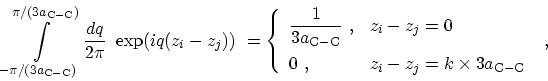(4.39)

where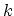is an integer number. One has to multiply the above result by a factor of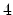, for the number of rings in the lattice period . Equation (4.39) justifies the approximation which only considers diagonal elements of the electron-phonon self-energy. As discussed in Section 4.3, by employing the nearest neighbor tight-binding method (block) tri-diagonal matrices are achieved. Keeping only diagonal elements of the electron-phonon self-energy, the matrices remain (block) tri-diagonal. Therefore, an efficient recursive method (Appendix H) can be used to calculate the inverse matrices. This implies considerable reduction of computational cost and memory requirement.

Using the result of (4.39) and the relations (2.15) and (2.19) the self-energy due to scattering with optical phonons can be written as(4.40)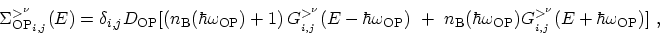(4.41)

where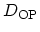is given by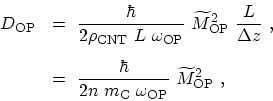(4.42)

where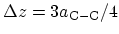(see (4.3)). In the second line in (4.42) the mass density of a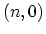zigzag CNT has been replaced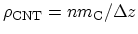, where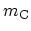is the mass of a carbon atom.

The retarded self-energy can be calculated as (3.76)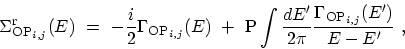(4.43)

where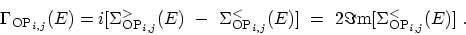(4.44)

Since the lesser and greater self-energies are assumed to be diagonal the retarded self-energy is also diagonal.

M. Pourfath: Numerical Study of Quantum Transport in Carbon Nanotube-Based Transistors